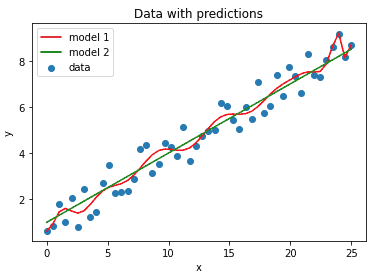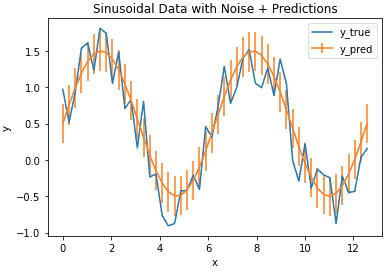# Metrics

This category groups posts on metrics used in machine learning. Each post focuses on a specific metric. The emphasis is on understanding how these tools actually work at a technical level. Here you will learn how to use machine learning metrics so that you can properly assess, and interpret, your models performance.## Bias and Variance

Bias and Variance Introduction Machine learning models are developed primarily to produce good predictions for some desired quantity. What we want is a model that can generalise well the patterns in our data in order to make reasonable predictions. Two important measures of how well a model generalises are bias and variance: Bias is the tendency …## Mean Absolute Error

Mean Absolute Error Introduction With any machine learning project, it is essential to measure the performance of the model. What we need is a metric to quantify the prediction error in a way that is easily understandable to an audience without a strong technical background. For regression problems, the Mean Absolute Error (MAE) is just such …## Implement the Bootstrap Method in Python from Scratch

Implement the Bootstrap Method in Python from Scratch In this post we will implement the Bootstrap Method, and use it to analyse a linear regression model. Through this exercise, we will understand how this technique works, and how you can apply the bootstrap method in Python from scratch. Motivation to Implement the Bootstrap Method A …